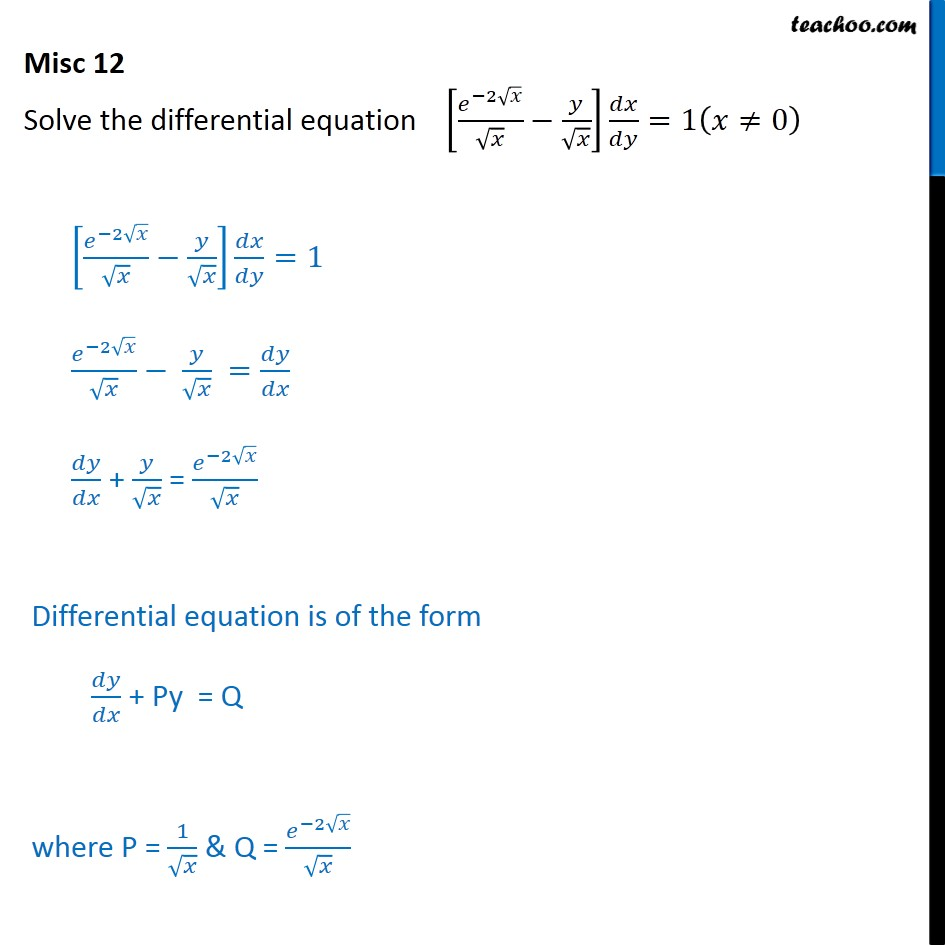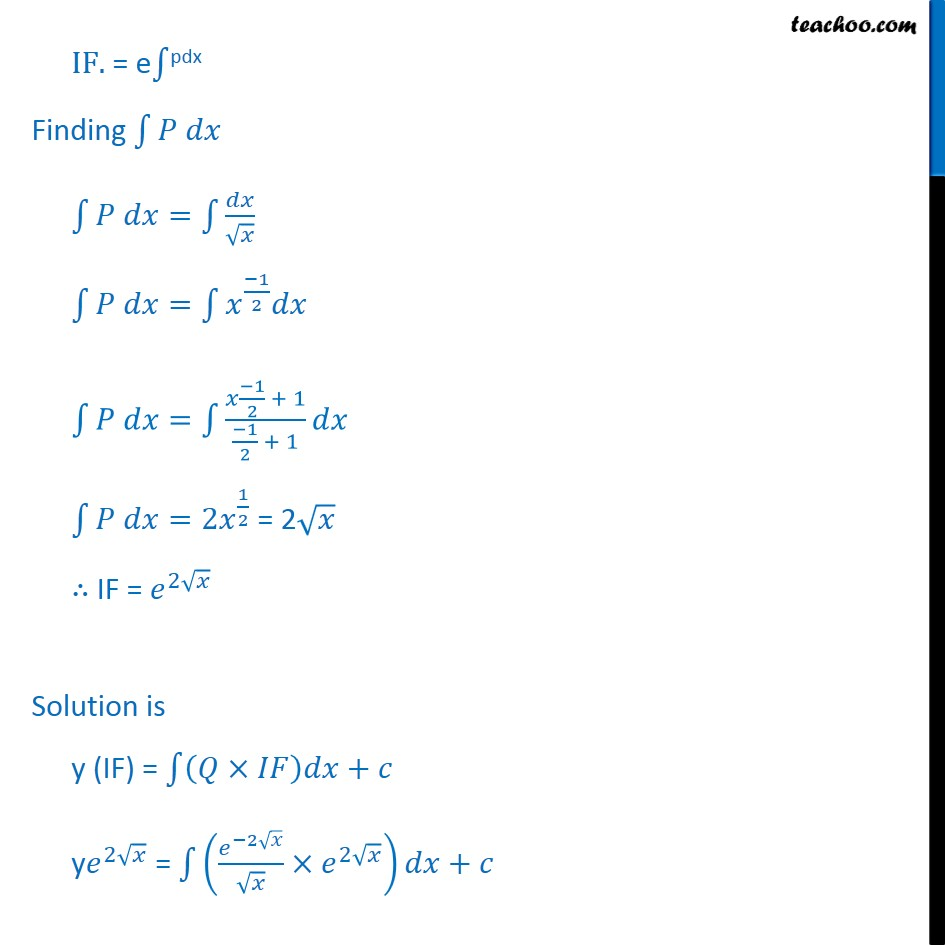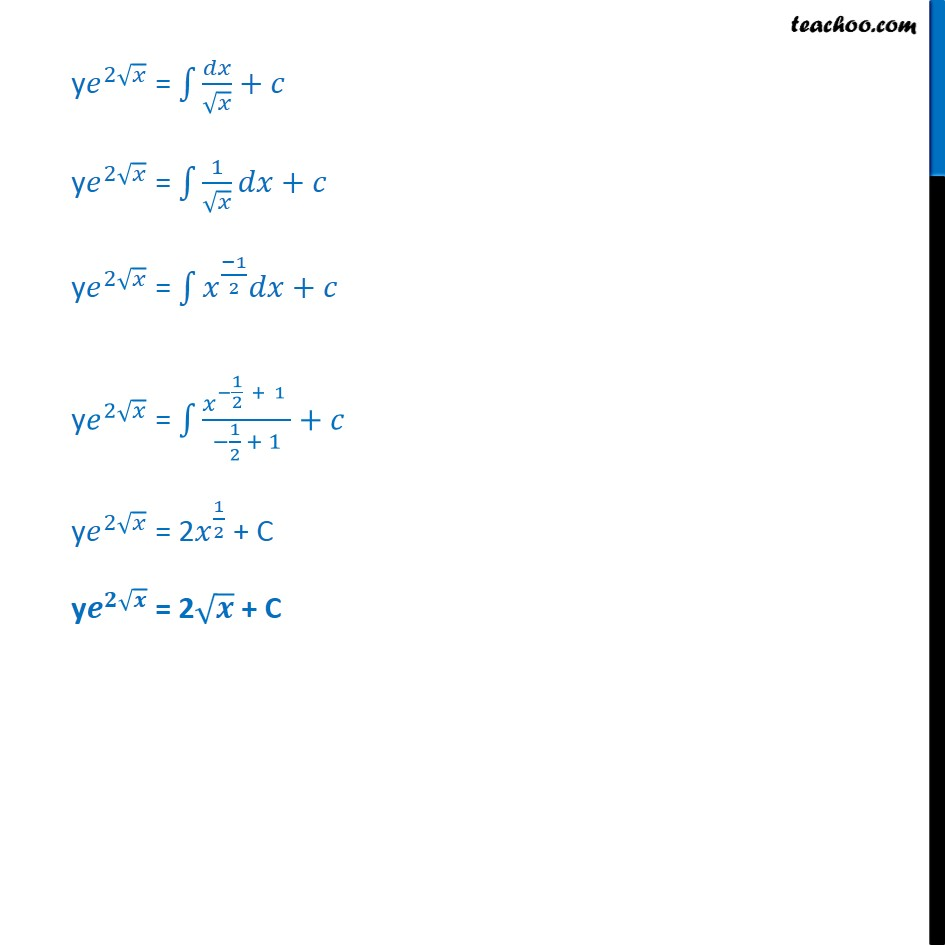Miscellaneous

Chapter 9 Class 12 Differential Equations
Serial order wiseLearn in your speed, with individual attention - Teachoo Maths 1-on-1 Class

### Transcript

Misc 10 Solve the differential equation 2 =1 0 2 =1 2 = + = 2 Differential equation is of the form + Py = Q where P = 1 & Q = 2 IF. = e pdx Finding = = 1 2 = 1 2 + 1 1 2 + 1 =2 1 2 = 2 IF = 2 Solution is y (IF) = + y 2 = 2 2 + y 2 = + y 2 = 1 + y 2 = 1 2 + y 2 = 1 2 + 1 1 2 + 1 + y 2 = 2 1 2 + C y = 2 + C